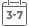# Computational Colour Science using MATLAB

• Publish Date: 2004-04-26
• Binding: Hardcover
• Author: Stephen Westland;Caterina Ripamonti
• Sale
• \$23.55
• Regular price \$109.31Presenting apractical, problem-based approach to colour physics, this titledescribes the key issues encountered in modern colour engineering, including efficient representation of colour information, fourier analysis of reflectance spectra and advanced colorimetric computation. Emphasis is placed on the practical applications rather than the techniques themselves, with material structured around key topics, such as colour calibration of visual displays, computer recipe prediction and models for colour-appearance prediction.

Each topic is carefully introduced at three levels to enhance student understanding. Firstly, theoretical ideas and background information are discussed, explanations of mathematical solutions then follow and finally practical solutions are presented using MATLAB.

• Includes a compendium of equations and numerical data required by the modern colour and imaging scientist.
• Numerous examples of solutions and algorithms for a wide-range of computational problems in colour science.
• Provides example scripts using the MATLAB programming language.

This text is a must-havefor students taking courses in colour science, colour chemistry and colour physics as well as technicians and researchers working in the area.

MORE FROM THIS COLLECTION